# The Azimuth Project Blog - cospans, wiring diagrams, and the behavioural approach

Joint post by John Baez and Brendan Fong.

Last time we heard about understanding types of networks as categories of decorated cospans.

• Decorated cospans post.

Earlier, David Spivak also told us about understanding networks as algebras over an operad.

Both these frameworks work at capturing notions of modularity and interconnection. Are they then related? How? In this post we want to discuss some similarities between decorated cospan categories constructed from functors with domain $(\mathrm{Set},+)$, and algebras for Spivak’s operad of wiring diagrams. The main idea is that the two approaches are ‘essentially’ equivalent, but that compared to decorated cospans, Spivak’s operad formalism puts greater emphasis on the distinction between the Frobenius morphisms and other morphisms in our category. The precise details are still to be worked out – jump in and help us!

### Operads

We begin with a bit about operads in general. Recall that an operad is similar to a category, except that instead of a set $\mathrm{hom}(x,y)$ of morphisms from any object $x$ to any object $y$, you have a set $\mathrm{hom}(x_1,...,x_n;y)$ of operations from any finite list of objects $x_1,...,x_n$ to any object $y$. If we have an operation $f \in \mathrm{hom}(x_1,...,x_n;y)$, we can call $x_1, \dots, x_n$ the inputs of $f$ and call $y$ the output of $f$.

We can compose operations in an operad. To understand how, it’s easiest to use pictures. We draw an operation in $\mathrm{hom}(x_1,...,x_n;y)$ as a little black box with $n$ wires coming in and one wire coming out: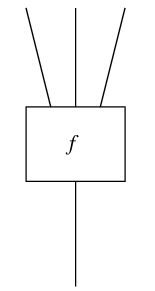The input wires should be labelled with the objects $x_1, \dots, x_n$ and the output wire with the object $y$, but I haven’t done this.

We are allowed to compose these operations as follows: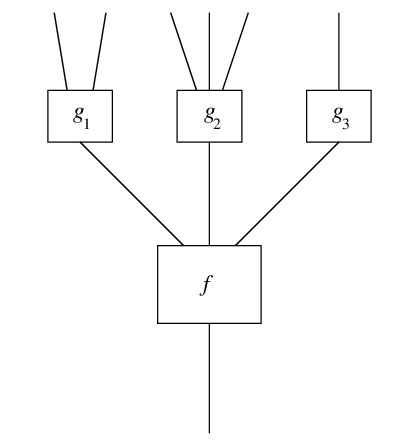as long as the outputs of the operations $g_1,\dots,g_n$ match the inputs of the operation $f$. The result is a new operation which we call $f \circ (g_1,\dots,g_n)$.

We demand that there be unary operations $1_x \in hom(x,x)$ serving as identities for composition, and we impose an associative law that makes a composite of composites like this well-defined: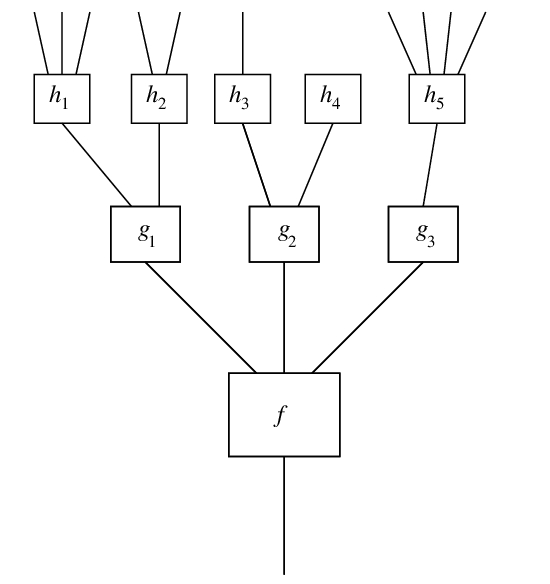So far this is the definition of a planar operad. In a full-fledged permutative operad, often called just an operad, we can also permute the inputs of an operation $f$ and get a new operation: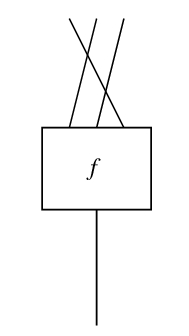which we call $f \sigma$ if $\sigma$ is the the permutation of the inputs. We demand that $(f \sigma) \sigma' = f (\sigma \sigma')$. And finally, we demand that permutations act in a way that is compatible with composition. For example: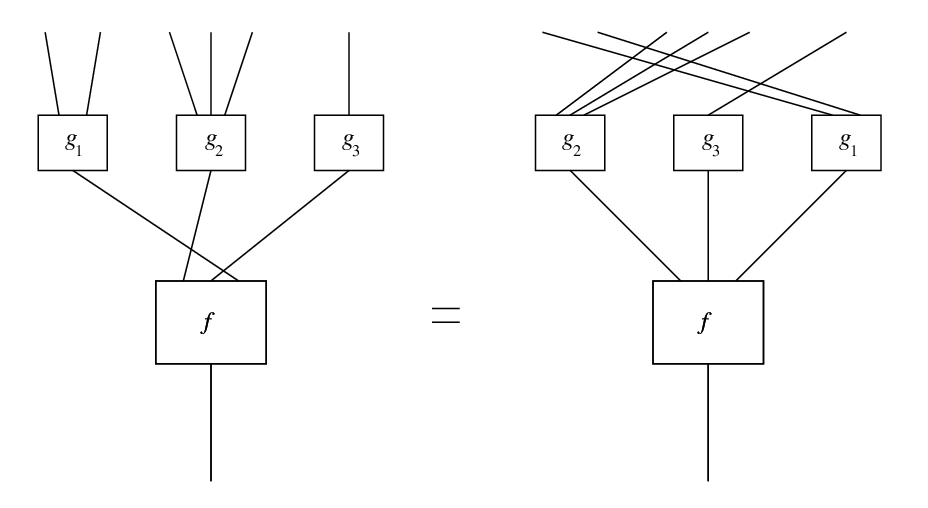Here we see that $(f \sigma) \circ (g_1, \dots, g_n)$ is equal to some obvious other thing. There is also a law saying $f \circ (g_1 \sigma_1, \dots, g_n \sigma_n)$ is equal to some other expression.

Operads are similar to symmetric monoidal categories. The idea is that in a symmetric monoidal category you can just form the tensor product $x_1 \otimes \dots \otimes x_n$ and talk about the set of morphisms $x_1 \otimes \dots \otimes x_n \to y$. Indeed any symmetric monoidal category gives an operad in this way: just define $\mathrm{hom}(x_1,...,x_n;y)$ to be $\mathrm{hom}(x_1 \otimes \cdots \otimes x_n, y)$. If we do this with Set, which is a symmetric monoidal category using the usual cartesian product of sets, we get an operad called $\mathrm{Sets}$.

An algebra for an operad $O$ is an operad homomorphism $O \to \mathrm{Sets}$. I know I haven’t said what an operad homomorphism is, but you can probably figure it out yourself. The point is this: an algebra for $O$ turns the abstract operations in $O$ into actual operations on sets!

### The operad of wiring diagrams

Spivak’s favorite operad is the operad of wiring diagrams. The operad of wiring diagrams $WD$ is an operad version of $\mathrm{Cospan}(\mathrm{FinSet})$, constructed in the vein suggested above: the objects are finite sets, and the morphisms from a set of sets $\{X_1,...,X_n\}$ to a set $Y$ are cospans $X_1+...+X_n \to Y$. Spivak draws these things as a big circle with $n$ small circles cut out from the interior. The outside of the big circle has $Y$ terminals marked on it, and the small circles each have $X_i$ terminals marked respectively. Then in the interior of this shape there are wires connecting these terminals. These are the so-called wiring diagrams.

You compose these wiring diagrams by pasting other wiring diagrams into each of the small circles.

The relationship with our Frobenius algebra diagrams is pretty simple: we draw our ‘wiring diagrams’ $X \to Y$ in a square, with the $X$ terminals on the left and $Y$ terminals on the right. To get a Spivak-approved wiring diagram, glue the top and bottom edges of this square together, then flatten the cylinder you get down into an annulus, with the $X$-side on the inside and $Y$-side on the outside. If $X = X_1+X_2$ you can imagine gluing opposite edges of the inside circle together to divide it into two small circles accordingly, and so on.

So loosely, we could think of the operad of wiring diagrams as like the PROP for special commutative Frobenius monoids.

### Relational algebras of type A

Algebras for wiring diagrams tell you what components you have available to wire together with your diagrams. An algebra for the operad of wiring diagrams is a functor $WD \to Sets$. What does this look like? Just like a functor for categories, it assigns to each natural number a set, and each wiring diagram a function.

In work related to decorated cospans (such as our draft paper on circuits or John and Jason Erbele’s work on signal flow diagrams), our semantics usually is constructed from a field of values: like assigning a velocity, or potential, or current to a variable. This gives us vector spaces and bimonoids and a bunch of nice structure. Spivak works more generally: he’s interested in the structure when you just have a set of values. While this means we can’t do some of the nice things we could do with a field, it also means this framework can do things like talk about logic gates, where the variables are boolean ones, or number theoretic questions, where you’re interested in the natural numbers.

So to discuss semantics we pick a set A of values, such as the real numbers or natural numbers or booleans or colours. We imagine then associating elements of this set to each wire in a wiring diagram. More technically, the algebra $\mathrm{Rel}A: WD \to \mathrm{Sets}$ then maps each finite set $X$ to the power set $\mathcal{P}(A^X)$ of the set $A^X$ of functions $X \to A$.

On the morphisms (the wiring diagrams themselves), this functor behaves like our copying/comparing Frobenius algebra (the white one in the signal flow calculus). Note that a function ($X \to A$) can be thought of as an ‘X-vector’ $(a_1,...,a_x)$ of ‘$A$-coordinates’. A wiring diagram $X \to Y$ is just a cospan $X \to N \leftarrow Y$ in $Set$, so it can be thought of as some compares ($X \to N$) followed by some copies ($N \to Y$). Thus, given a wiring diagram $X \to Y$, we can consider a partial function that maps an $X$-vector to the $Y$-vector by doing these compares, and if it passes them does the copies and returns the resulting Y-vector, but if not returns ‘undefined’. We can then define a map $\mathcal{P}(A^X) \to \mathcal{P}(A^Y)$ which takes a set of $X$-vectors to its image under this partial function.

This semantics is called the relational $WD$-algebra of type $A$. We can think of it as like the white fragment of the signal flow calculus.

Note that we can’t do the black signal flow calculus, because we only have a set $A$ of values, not a field $A$, and the black signal flow calculus requires addition.

### Operads and the behavioural approach

In formulating Frobenius monoids this way, Spivak achieves something that we’ve been working hard to find ways to acheive: a separation of the behavioural structure from the interconnection structure.

What do I mean by this? In his ‘behavioural approach’, Willems makes the point that for all their elaborate and elegant formulation, in the end physical laws just come down to dividing the set of what might a priori be possible (the ‘universum’) into the set of things that actually are possible (the ‘behaviour’), and the set of things that aren’t). Here the universum is the set $A^X$: a priori, on each of the wires in $X$, we might associate any value of $A$. For example, to the two wires at the ends of a resistor, we might a priori associate any pair of currents. But physical law, here Kirchhoff’s current law, says otherwise: the currents must be equal and opposite. So the ‘behaviour’ is the subset $(i,-i)$ of the universum $\mathbb{R}^2$.

So you can say that to each object $X$ in the operad of wiring diagrams the relational algebra of type $A$ associates the set $\mathcal{P}(A^X)$ of possible behaviours – the universum is $A^X$. ($\mathcal{P}(A^X)$ forms some sort of meta-universum, where you can discuss physical laws about physical laws, commonly called ‘principles’.)

The second key aspect of the behavioural approach is that the behaviours of larger systems can be constructed from the behaviours of its subsystems, if we understand the so-called ‘interconnection structure’ well enough. This is a key principle in engineering: we build big, complicated systems from a much smaller set of components, whether it be electronics from resistors and inductors, or mechanical devices from wheels and rods and ropes, or houses from Lego bricks. The various interconnection structures here are the wiring diagrams, and our relational algebras say they act by what Willems calls ‘variable sharing’.

This division between behaviour and interconnection motivates the decorated cospans construction (the decorations are the ‘components’, the cospans the ‘interconnection’) and multigraph categories (morphisms = ‘components’, Frobenius monoids = ‘interconnection’).

So it’s good to have this additional way of thinking about things in our repertoire: Operads describe ‘interconnection’, their algebras ‘behaviours’.

The separation Spivak achieves, however, seems to me to come at the cost of neat ways to talk about individual components, and perhaps this can be seen as the essential difference between the two approaches. By including our components as morphisms, we can talk more carefully about them and additional structure individual components have. On the other hand, by lumping all the components into the objects, Spivak can talk more carefully about how the interconnection structure acts on all behaviours at once.

### Other operads of wiring diagrams

One advantage of the operad approach is that you can easily tweak your operad to talk about different sorts of network structure. Sometimes you can make similar adjustments with decorated cospans too, such as working over the category of typed finite sets, rather than just finite sets, to discuss networks in which wires have types, and only wires of the same types can be connected together. A physical example is a model of a hydroelectric power plant, where you don’t want to connect a water pipe with an electrical cable! This is also a common technique in computer science, where you don’t want to try to multiply two strings of text, or try to interpret a telephone number as a truth value.

But some modifications are harder to do with decorated cospans. In some other papers, Spivak employs a more restricted operad of wiring diagrams, in which joining wires and terminating wires is not allowed, among other things. He uses this to formalise graphical languages for certain types of discrete-time processes, open dynamical systems, including mode-dependent ones.

More detail:

B. Fong - Decorated cospans. http://arxiv.org/abs/1502.00872.

D. Spivak - The operad of wiring diagrams: formalizing a graphical language for databases, recursion, and plug-and-play circuits. http://arxiv.org/abs/1305.0297.